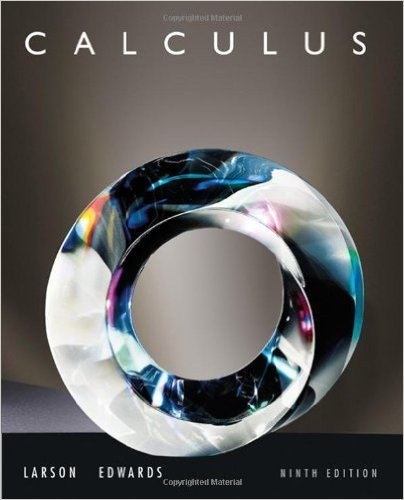×
×

# Solutions for Chapter 13.4: Differentials## Full solutions for Calculus | 9th Edition

ISBN: 9780547167022Solutions for Chapter 13.4: Differentials

Solutions for Chapter 13.4
4 5 0 315 Reviews
25
0
##### ISBN: 9780547167022

This textbook survival guide was created for the textbook: Calculus , edition: 9. This expansive textbook survival guide covers the following chapters and their solutions. Since 48 problems in chapter 13.4: Differentials have been answered, more than 64305 students have viewed full step-by-step solutions from this chapter. Calculus was written by and is associated to the ISBN: 9780547167022. Chapter 13.4: Differentials includes 48 full step-by-step solutions.

Key Calculus Terms and definitions covered in this textbook
• Base

See Exponential function, Logarithmic function, nth power of a.

• Bounded above

A function is bounded above if there is a number B such that ƒ(x) ? B for all x in the domain of ƒ.

• Cone

See Right circular cone.

• Directrix of a parabola, ellipse, or hyperbola

A line used to determine the conic

• Equilibrium price

See Equilibrium point.

• Focal axis

The line through the focus and perpendicular to the directrix of a conic.

• Half-life

The amount of time required for half of a radioactive substance to decay.

• Logistic growth function

A model of population growth: ƒ1x2 = c 1 + a # bx or ƒ1x2 = c1 + ae-kx, where a, b, c, and k are positive with b < 1. c is the limit to growth

• Multiplication principle of probability

If A and B are independent events, then P(A and B) = P(A) # P(B). If Adepends on B, then P(A and B) = P(A|B) # P(B)

• Number line graph of a linear inequality

The graph of the solutions of a linear inequality (in x) on a number line

• Obtuse triangle

A triangle in which one angle is greater than 90°.

• Odd-even identity

For a basic trigonometric function f, an identity relating f(x) to f(-x).

• Position vector of the point (a, b)

The vector <a,b>.

• Quotient identities

tan ?= sin ?cos ?and cot ?= cos ? sin ?

• Random numbers

Numbers that can be used by researchers to simulate randomness in scientific studies (they are usually obtained from lengthy tables of decimal digits that have been generated by verifiably random natural phenomena).

• Relation

A set of ordered pairs of real numbers.

• Shrink of factor c

A transformation of a graph obtained by multiplying all the x-coordinates (horizontal shrink) by the constant 1/c or all of the y-coordinates (vertical shrink) by the constant c, 0 < c < 1.

• Solve by elimination or substitution

Methods for solving systems of linear equations.

• Variable

A letter that represents an unspecified number.

• Ymin

The y-value of the bottom of the viewing window.

×